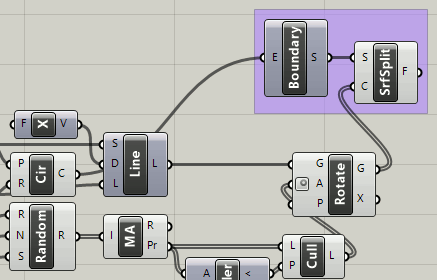# Dividing a circular surface into different parts

I would like to divide a circular surface into different parts, I would like to be able to parametracize this, meaning to say that I can control the sizes of each individual pie surface.

Thank you!

1 Like

Hi,

Thank you for your quick response but I would like to know, because this will only be creating lines from the center of the circle to the different points along the curve within the circle, how can i create surfaces from this divided parts?

Thank you!5 Likes

Hi,

Thank you for your help, but could I ask is it possible to divide the circle as a curve into different segments. The divide curve function results in multiple points, however, I would like to extract the divided curves individually as well.

Thank you

Hi, as I am fairly new to grasshopper i kinda got stuck at every juncture, I understood the line rotations, but I did not know how to shatter it, as the rotation outputs geometries however the shatter function requires a parameter. And I did not know what a parameter meant in this context.

How does the curve closest point work here? I understand that what you are doing is essentially extracting the end point of the curve so if I were to use a curve on point function, that would result in locating that point, but why must it be a parameter and not the position of the point?

Thank you for your help anyway!